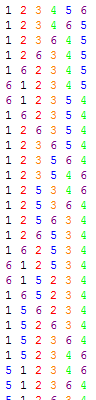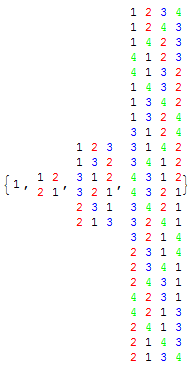# Campanology

It is usual, in Cambridge, to hear a plethora of bells chiming. Being within hearing range of the chapels of both Trinity and King’s college, I am greeted with clocks chiming every quarter-hour.

On Sunday mornings, however, someone plays the entire full peal (or extent) on six bells. This involves ringing them in all 6! = 720 possible permutations, in sequence, to create a beautiful sound lasting for about half an hour. Here are the first few permutations:Due to both aesthetic and practical considerations, however, not all of the (6!)! possible sequences of permutations are admissible. Generally, the following restrictive set of rules must be obeyed:

• Two adjacent permutations must be precisely one transposition (2-cycle) away from each other;
• This 2-cycle must permute two adjacent bells.

This ensures that the time delay between the same bell ringing twice is either 5, 6 or 7 intervals. The way that bell-ringers achieve this is to use the recursive Steinhaus-Johnson-Trotter algorithm. We begin with a valid sequence on n-1 bells, and extend it to a valid sequence on n bells. By induction from the trivial base case, this leads to sequences on any number of bells, including six.The trivial base case is the single permutation of one bell. We replace every permutation on n-1 bells with n permutations on n bells. For example, the permutation {a,b,c,d} is replaced with:

• {a,b,c,d,e}
• {a,b,c,e,d}
• {a,b,e,c,d}
• {a,e,b,c,d}
• {e,a,b,c,d}

This is the same permutation with ‘e’ inserted at every possible position in order. For the next permutation, we reverse the positions where we insert the ‘e’. For instance, if the next permutation is {a,b,d,c}:

• {e,a,b,d,c}
• {a,e,b,d,c}
• {a,b,e,d,c}
• {a,b,d,e,c}
• {a,b,d,c,e}

It is clear to see why the algorithm works as intended. It is also a proof that every permutation can be reached by swapping adjacent elements, and thus that any 2-cycle together with any p-cycle generates the symmetric group S_p.

Similarly recursive algorithms are used to generate the sequence of moves for solving the Tower of Hanoi puzzle.

This entry was posted in Uncategorized. Bookmark the permalink.